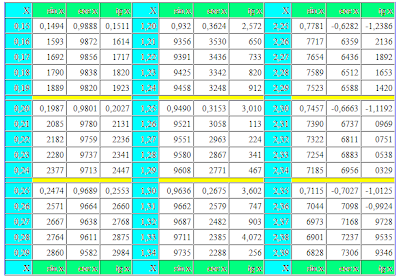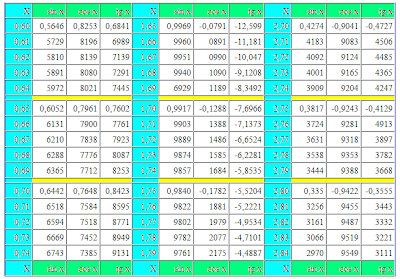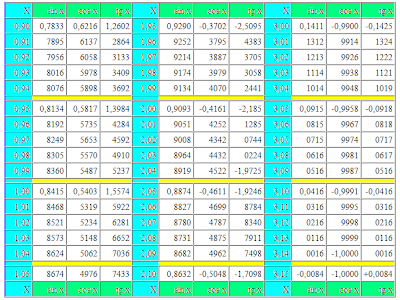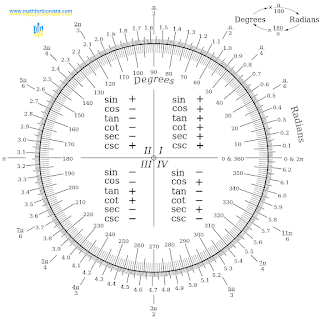## 2/14/2012

This trigonometric table is made for the values of corners in radians. Radians are here given as decimal fractions within two signs after a comma. Value of sine, cosine and tangent given within four signs after a comma. It is such small trigonometric table in radians. You can see the picture Degree-Radian Conversion at the end of the page.

## Table of trigonometric values in degrees:sin costan cotIn this trigonometric table the value of corner in radians closes on a 3,15 radian, that corresponds hardly anymore 180 degrees in the degree measure of corners. Here you will not find the value of tangent, equal to unit, value of sine, equal to unit and value of cosine, equal to the zero. In the radian measure of corners to get these values unassisted number of Pi it is impossible. And as a self number of Pi is an endless shot not having the exact meaning, expediency of goniometry in radians is very doubtful. Radians - it, put it mildly, strange unit of measurement.

Values of corner in radians are in blue columns mark the letter of "X". In three columns the values of sin x are given on the right, cos x and tg x for corners in radians. Value of cotangent, secant and cosecant to the table not driven, as these trigonometric functions are reverse shots to driven to the table. For the receipt of values of ctg x, sec x and cosec x in radians, it is needed to divide unit into a tangent, cosine or sine of corresponding corner in radians.Degree-Radian Conversion

1.1.